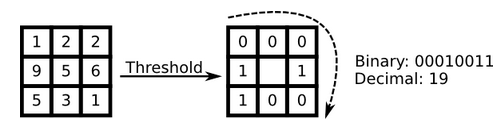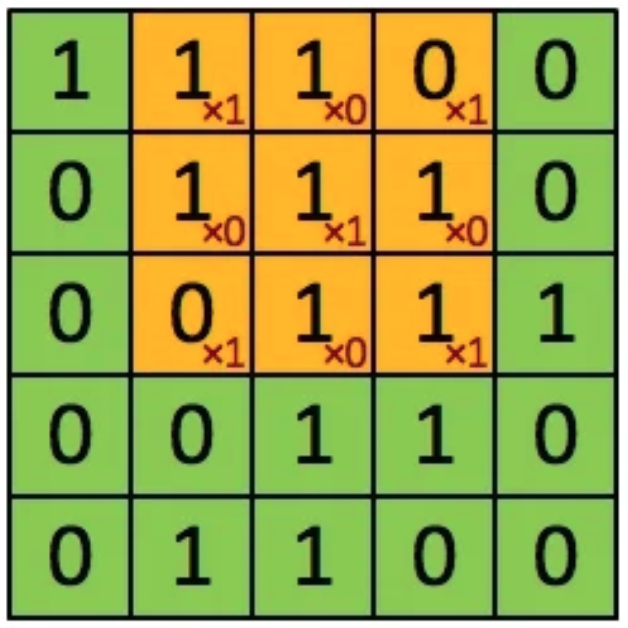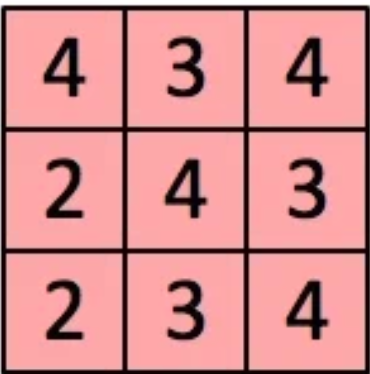# Face Recognition in Production

## How it works in large-scale systems

Posted by Xiaozhe Yao on April 18, 2020

## Definition and terms

In many different types of software, face recognition plays an important role. Typically, the recognition process includes the following steps:

• Face Detection. Face detection tries to find a square or a circle in an image. The computational complexity for face detection is rather low and can be performed really fast (up to 2000 frames per second on a modern CPU, source: GitHub - ShiqiYu/libfacedetection: An open-source library for face detection in images. The face detection speed can reach 1000FPS.). With face detection, we could narrow our region of interests, so that we do not need to perform recognition in every area of an image, where most of which do not contain any faces.
• Face Alignment. Or key structure extraction. It is used to extract the geometric structure of components, such as eyes, mouths, etc. The result of face alignment is an array of coordinates to indicate where the components are.
• Face Representation. It converts a face image into a dense vector. Ideally, faces belong to a single person should be mapped to the same vector.
• Face Matching: Verification or recognition. Verification is 1:1 comparison to check if two faces belong to the same person. Recognition is 1:N comparison to know which person in the database does the face belong to.

From the machine learning side, face recognition can be regarded as a classification task. Though classification task has been thoroughly investigated and there are lots of existing algorithms to it, it’s still a huge challenge for face recognition due to several significant challenges:

• Face recognition task usually includes millions of thousands of labels. For example, in Alipay and iPhone, which are both used by billions of users, have tons of labels of faces.
• Face recognition requires the algorithms to be performed in seconds, not hours. In some cases, it requires the algorithm to be near real-time.
• Not all labels are provided as it is. In production, there are always new labels to come in and requires the algorithms to be evolvable with the new dataset.
• Face itself is challenging. Unlike text analysis and some other tasks, faces-in-the-wild is highly variable, due to the changes in illumination conditions, ages changes, wearing a mask or not, etc.
• The base dataset may include wrongly-labelled data, which usually occurs in this scenario and can not be avoided easily (Programmers are unable to check if the data is correctly labelled).

## History of face recognition techniques

During the development of face recognition, there are three milestones.

• Eigenfaces.
• Hand-crafted features.
• A convolutional neural network as a feature extractor.

### Eigenfaces

The main idea of eigenfaces is to project high-dimensional face features into a lower-dimensional vector, and then classify images with low-dimension vectors.

Eigenfaces is a set of "general faces". Theoretically, any face can be regarded as a weighted sum of eigenfaces, for example,

obama_face=0.25*eigen_1 + 0.3*eigen_2 - 0.4*eigen_3

The process usually includes:

• Collecting training dataset. Each image, as a matrix $(r \times c)$, will be re-shaped to a vector $1\times(rc)$. Then all images can be seen as a $n\times(rc)$ matrix $T$.
• Then we calculate the average face vector and normalize every face in the database to be $face_i-avg_f$. Then we will have a new, normalized matrix. With this matrix, we can then compute the covariance matrix $S$, and then eigenvalues and eigenvectors of S. We will then have $n$ eigenvectors (faces).
• We can then perform PCA (Principal Component Analysis) on the eigenvectors to find the primary component.
• When a new face comes in, we convert it into a vector, project it into eigenface space, and we can then calculate the distance between the new face and the face space to check if it is a face (detection). If it is a face, we then calculate its weights, as in the example, it will be $(0.25,0.3,0.4)$. By comparing the weights with existing faces, we will be able to know if two faces belong to the same person, or which person does the face belong to.

### Hand-crafted features

In the eigenface method, we find that faces, of the same person, under different illumination conditions, can be looked very differently. That makes the method vulnerable to illumination variations. To tackle the problem, researchers designed several features to extract from images and use these features as low-dimensional vectors to compare. One of which is Local Binary Pattern (LBP). Theoretically LBP can avoid the variations in different light conditions.

The extraction of LBP feature works as below:• We take a $3\times3$ window from the image (and use sliding window to walk over the whole image). Then we take the centre as the threshold of the region and compare it with other point. If the value is larger than the threshold, assign it to be $1$, otherwise $0$. With these we will get a 8-bit number, i.e. 256 different patterns. We then use this value to reflect the pattern of this window. To be formal, $LBP(x_c,y_c)=\sum_{p=0}^{p-1}2^pSign(i_p-i_c)$.

• Then similar to eigen face, we can compare new faces with exisiting patterns.

Ideally, the influence of light, on a small patch, will perform similarly on all pixels. i.e. In different illumnation conditions, every pixel in a same patch will (ideally) add a same constants $c$. As seen in the above process, it won't change anything. Experiments showed that LBP and other hand-crafted features work better than Eigenface method. However, in real life, the assumptions should be carefully examined.

### Deep Neural Networks

Even though hand-crafted features have performed pretty well on face recognition tasks, there's still spaces to improve, and the approach is pretty clear: As hand-crafted features work pretty well, can computers help us design features automatically from the dataset?

#### Neural Networks

I am not going to introduce vanilla neural networks. Please let me know if it is needed. I'd rather describe some important improvements of CNN over other techniques.

Neural networks are designed to perform tasks by taking examples and exisiting data. In designing a neural network, we need to know what kind of architectures fit our needs and our optimization goals.

##### Architecture - CNNConvolutional neural network is a deep learning architecture, which usually works on images.

Convolution layer is the key component of CNN, which computes and extracts the convolved feature from an image. For example, assume we have a local region of an image as a $5\times5$ matrix, and we have a kernel $(1,0,1;0,1,0;1,0,1)$.We can then calculate the local convolved feature asAs we seen in the above process, CNN uses local operations, which performed on local pixels. With the previous knowledge we know the neighboring pixels are more important for a single pixel rather than a random pixel on the image. Thus it is very useful to compare pixels in local regions, rather than the global. The nature in convolutional neural network followed the pattern of images and is the architecture that we want. Besides of this nature, it is also more efficient than fully connected networks as it shares weights along many different neurons.

In face recognition, we want the output of our network to be a vector that satifies:

• For a same person, the distances between vectors are as near as possible.

• For different persons, the distance between vectors are as far as possible.

This requirements is somehow an "embedding". In general image classification tasks, we use softmax loss, which have no requirement for the distance. Therefore, researchers proposed center loss, which add another loss to original softmax loss that calculate the center point of every class, and the distance of each vector to the center point. With center loss, we can force the neural network to learn a representation that minimize the distance inside a class, and maximize the distance across different classes.